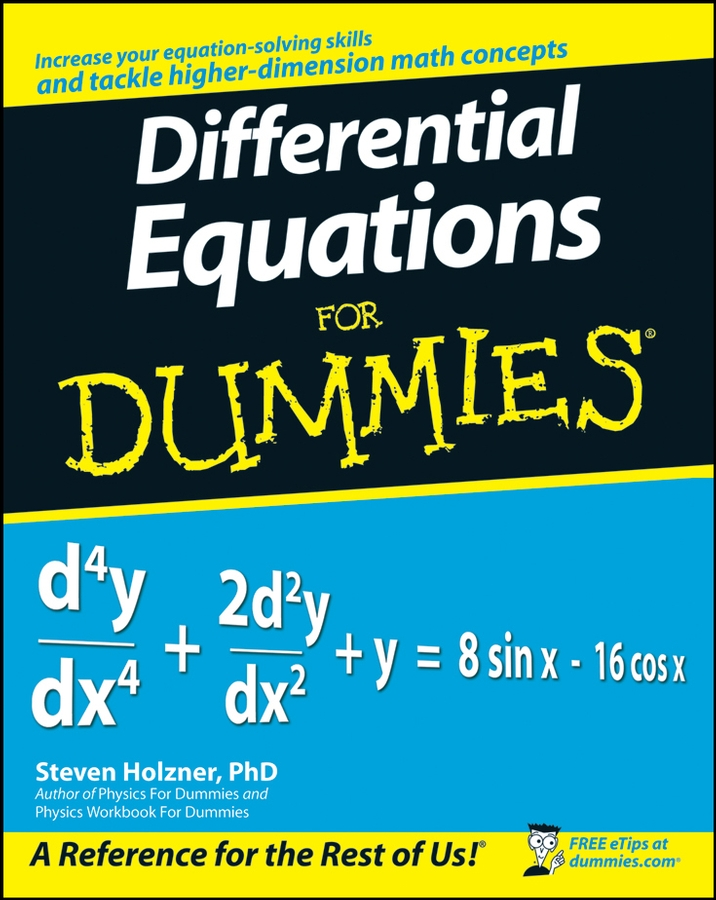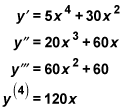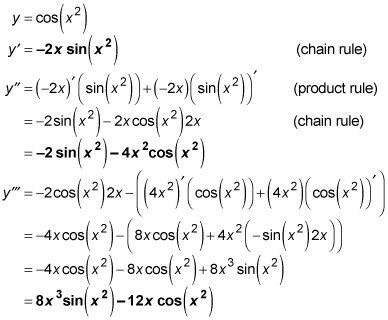##### Differential Equations For DummiesIn calculus, you often need to take higher order derivatives — that is, the derivative of a derivative, or the derivative of a derivative of a derivative, and so on. Why? Well, for example, a second derivative tells you the acceleration of a moving body.

So how do you do this? Simple! To find a higher order derivative, you just treat the first derivative as a new function and take its derivative in the ordinary way. You can keep doing this indefinitely. (Well, if you want to.)

The following practice questions won't ask you to go on indefinitely, but they will ask you to find third and fourth derivatives.

## Practice questions

1. For y = x5 + 10x3, find the 1st, 2nd, 3rd, and 4th derivatives.

2. For y = cos (x2), find the 1st, 2nd, and 3rd derivatives.

## Answers and explanations

1. For y = x5 + 10x3, the 1st, 2nd, 3rd, and 4th derivatives are as follows:2. For y = cos (x2), the 1st, 2nd, and 3rd derivatives are as follows: